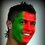# Integration

$\large \int \dfrac{\arctan(x)}{x} \, dx$ Can anyone tell how this integration can be done?Note by Pratik Roy
5 years, 3 months ago

This discussion board is a place to discuss our Daily Challenges and the math and science related to those challenges. Explanations are more than just a solution — they should explain the steps and thinking strategies that you used to obtain the solution. Comments should further the discussion of math and science.

When posting on Brilliant:

• Use the emojis to react to an explanation, whether you're congratulating a job well done , or just really confused .
• Ask specific questions about the challenge or the steps in somebody's explanation. Well-posed questions can add a lot to the discussion, but posting "I don't understand!" doesn't help anyone.
• Try to contribute something new to the discussion, whether it is an extension, generalization or other idea related to the challenge.

MarkdownAppears as
*italics* or _italics_ italics
**bold** or __bold__ bold
- bulleted- list
• bulleted
• list
1. numbered2. list
1. numbered
2. list
Note: you must add a full line of space before and after lists for them to show up correctly
paragraph 1paragraph 2

paragraph 1

paragraph 2

[example link](https://brilliant.org)example link
> This is a quote
This is a quote
    # I indented these lines
# 4 spaces, and now they show
# up as a code block.

print "hello world"
# I indented these lines
# 4 spaces, and now they show
# up as a code block.

print "hello world"
MathAppears as
Remember to wrap math in $$ ... $$ or $ ... $ to ensure proper formatting.
2 \times 3 $2 \times 3$
2^{34} $2^{34}$
a_{i-1} $a_{i-1}$
\frac{2}{3} $\frac{2}{3}$
\sqrt{2} $\sqrt{2}$
\sum_{i=1}^3 $\sum_{i=1}^3$
\sin \theta $\sin \theta$
\boxed{123} $\boxed{123}$

Sort by:

you need knowledge of euler's formula and polylogarithm. first we write $\tan(y)=x\to x=\arctan(x)=y$ or $x=\dfrac{\sin(y)}{\cos(y)}=-i\dfrac{e^{2iy}-1}{e^{2iy}+1}$ So $y=\dfrac{i}{2} \ln(1+ix)-\dfrac{i}{2} \ln(1-ix)$ so, $\arctan(x)=\dfrac{i}{2} \ln(1+ix)-\dfrac{i}{2} \ln(1-ix)$ putting this in the integral $I=\dfrac{i}{2}\int \dfrac{ \ln(1+ix)-\ln(1-ix)}{x}dx= \dfrac{i}{2}(li_2(-ix)-li_2(ix))+C$

- 5 years, 3 months ago

- 5 years, 3 months ago

from here try the integration tricks wikis and advance integration. while this is not complete yet the contents there are good enough for this problem.

- 5 years, 3 months ago

thanks

- 5 years, 3 months ago

how can i calculate the integral if the limits are given?

- 5 years, 3 months ago